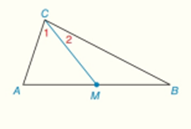Chapter 3.CT, Problem 8CT### Elementary Geometry for College St...

6th Edition
Daniel C. Alexander + 1 other
ISBN: 9781285195698

#### Solutions

Chapter
Section### Elementary Geometry for College St...

6th Edition
Daniel C. Alexander + 1 other
ISBN: 9781285195698
Textbook Problem
1 views

# C M ¯ is the median for Δ A B C from vertex C to side A B ¯ .a) Name two line segments that must be congruent.                                     _ b) Is ∠ 1 necessarily congruent to ∠ 2 ?                                     _

To determine

a)

To find:

The two congruent line segments.

Explanation

Definition:

A median to the line divides it in two equal parts.

Given:

The given figure is,

Figure (1)

And,

CM¯ is the median of ΔABC from vertex C to side AB¯

To determine

b)

To check:

The given congruency.

### Still sussing out bartleby?

Check out a sample textbook solution.

See a sample solution

#### The Solution to Your Study Problems

Bartleby provides explanations to thousands of textbook problems written by our experts, many with advanced degrees!

Get Started

#### Show that sinxxif0x2.

Calculus (MindTap Course List)

#### In Exercises 23-36, find the domain of the function. 23. f(x) = x2 + 3

Applied Calculus for the Managerial, Life, and Social Sciences: A Brief Approach

#### Explain the difference between a matched-subjects design and a repeated-measures design.

Essentials of Statistics for The Behavioral Sciences (MindTap Course List)

#### Evaluate the integral. 8. 3t2t+1dt

Single Variable Calculus: Early Transcendentals

#### The polar coordinates of the point P in the figure at the right are:

Study Guide for Stewart's Single Variable Calculus: Early Transcendentals, 8th

#### Describe the purpose of a dismantling design.

Research Methods for the Behavioral Sciences (MindTap Course List)